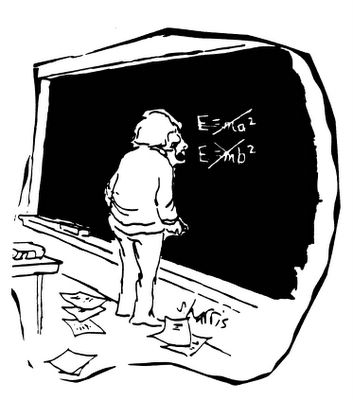## Wednesday, December 21, 2005

### E (Still) = mc2Cartoon © S. Harris

A direct test of E = mc². 2005. Rainville, J.K., et al. Nature 438: 1096-1097.

ABSTRACT: One of the most striking predictions of Einstein's special theory of relativity is also perhaps the best known formula in all of science: E=mc². If this equation were found to be even slightly incorrect, the impact would be enormous -- given the degree to which special relativity is woven into the theoretical fabric of modern physics and into everyday applications such as global positioning systems. Here we test this mass–energy relationship directly by combining very accurate measurements of atomic-mass difference, ∆m, and of γ-ray wavelengths to determine E, the nuclear binding energy, for isotopes of silicon and sulphur. Einstein's relationship is separately confirmed in two tests, which yield a combined result of 1-∆mc²/E=(-1.4±4.4)x10-7, indicating that it holds to a level of at least 0.00004%. To our knowledge, this is the most precise direct test of the famous equation yet described.

From the press release:

Albert Einstein was correct in his prediction that E=mc2, according to scientists at the Commerce Department's National Institute of Standards and Technology (NIST) and the Massachusetts Institute of Technology (MIT) who conducted the most precise direct test ever of what is perhaps the most famous formula in science.

The researchers added to a catalog of confirmations that matter and energy are related in a precise way. Specifically, energy (E) equals mass (m) times the square of the speed of light (c²), a prediction of Einstein's theory of special relativity. By comparing NIST measurements of energy emitted by silicon and sulfur atoms and MIT measurements of the mass of the same atoms, the scientists found that E differs from mc² by at most 0.0000004, or four-tenths of 1 part in 1 million. This result is "consistent with equality" and is 55 times more accurate than the previous best direct test of Einstein's formula, according to the paper.

Read the rest of the press release HERE